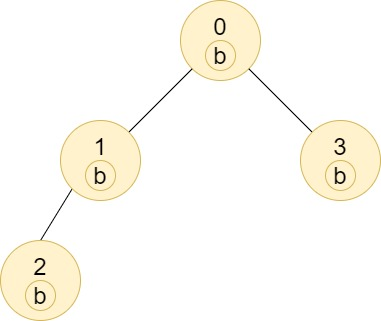Formatted question description: https://leetcode.ca/all/1519.html

# 1519. Number of Nodes in the Sub-Tree With the Same Label (Medium)

Given a tree (i.e. a connected, undirected graph that has no cycles) consisting of n nodes numbered from 0 to n - 1 and exactly n - 1 edges. The root of the tree is the node 0, and each node of the tree has a label which is a lower-case character given in the string labels (i.e. The node with the number i has the label labels[i]).

The edges array is given on the form edges[i] = [ai, bi], which means there is an edge between nodes ai and bi in the tree.

Return an array of size n where ans[i] is the number of nodes in the subtree of the ith node which have the same label as node i.

A subtree of a tree T is the tree consisting of a node in T and all of its descendant nodes.

Example 1:Input: n = 7, edges = [[0,1],[0,2],[1,4],[1,5],[2,3],[2,6]], labels = "abaedcd"
Output: [2,1,1,1,1,1,1]
Explanation: Node 0 has label 'a' and its sub-tree has node 2 with label 'a' as well, thus the answer is 2. Notice that any node is part of its sub-tree.
Node 1 has a label 'b'. The sub-tree of node 1 contains nodes 1,4 and 5, as nodes 4 and 5 have different labels than node 1, the answer is just 1 (the node itself).


Example 2:Input: n = 4, edges = [[0,1],[1,2],[0,3]], labels = "bbbb"
Output: [4,2,1,1]
Explanation: The sub-tree of node 2 contains only node 2, so the answer is 1.
The sub-tree of node 3 contains only node 3, so the answer is 1.
The sub-tree of node 1 contains nodes 1 and 2, both have label 'b', thus the answer is 2.
The sub-tree of node 0 contains nodes 0, 1, 2 and 3, all with label 'b', thus the answer is 4.


Example 3:Input: n = 5, edges = [[0,1],[0,2],[1,3],[0,4]], labels = "aabab"
Output: [3,2,1,1,1]


Example 4:

Input: n = 6, edges = [[0,1],[0,2],[1,3],[3,4],[4,5]], labels = "cbabaa"
Output: [1,2,1,1,2,1]


Example 5:

Input: n = 7, edges = [[0,1],[1,2],[2,3],[3,4],[4,5],[5,6]], labels = "aaabaaa"
Output: [6,5,4,1,3,2,1]


Constraints:

• 1 <= n <= 10^5
• edges.length == n - 1
• edges[i].length == 2
• 0 <= ai, bi < n
• ai != bi
• labels.length == n
• labels is consisting of only of lower-case English letters.

Related Topics:
Depth-first Search, Breadth-first Search

## Solution 1. DFS

// OJ: https://leetcode.com/problems/number-of-nodes-in-the-sub-tree-with-the-same-label/

// Time: O(N)
// Space: O(N)
class Solution {
vector<vector<int>> G;
vector<int> ans, seen;
vector<int> dfs(int u, string &labels) {
seen[u] = 1;
vector<int> cnt(26, 0);
for (int v : G[u]) {
if (seen[v]) continue;
auto sub = dfs(v, labels);
for (int i = 0; i < 26; ++i) cnt[i] += sub[i];
}
cnt[labels[u] - 'a']++;
ans[u] = cnt[labels[u] - 'a'];
return cnt;
}
public:
vector<int> countSubTrees(int n, vector<vector<int>>& E, string labels) {
G.assign(n, {});
ans.assign(n, 0);
seen.assign(n, 0);
for (auto &e : E) {
G[e].push_back(e);
G[e].push_back(e);
}
dfs(0, labels);
return ans;
}
};


Java

class Solution {
public int[] countSubTrees(int n, int[][] edges, String labels) {
Map<Integer, Set<Integer>> edgesMap = new HashMap<Integer, Set<Integer>>();
for (int[] edge : edges) {
int node0 = edge, node1 = edge;
Set<Integer> set0 = edgesMap.getOrDefault(node0, new HashSet<Integer>());
Set<Integer> set1 = edgesMap.getOrDefault(node1, new HashSet<Integer>());
edgesMap.put(node0, set0);
edgesMap.put(node1, set1);
}
Map<Integer, Integer> parentMap = new HashMap<Integer, Integer>();
Map<Integer, int[]> labelsMap = new HashMap<Integer, int[]>();
int[] levels = new int[n];
Arrays.fill(levels, -1);
levels = 0;
List<Integer> list = new ArrayList<Integer>();
Queue<Integer> queue = new LinkedList<Integer>();
queue.offer(0);
while (!queue.isEmpty()) {
int node = queue.poll();
int[] labelsCount = new int;
char label = labels.charAt(node);
labelsCount[label - 'a']++;
labelsMap.put(node, labelsCount);
int level = levels[node];
Set<Integer> nextNodes = edgesMap.getOrDefault(node, new HashSet<Integer>());
for (int nextNode : nextNodes) {
if (levels[nextNode] < 0) {
parentMap.put(nextNode, node);
levels[nextNode] = level + 1;
queue.offer(nextNode);
}
}
}
int[] counts = new int[n];
for (int i = list.size() - 1; i >= 0; i--) {
int node = list.get(i);
char label = labels.charAt(node);
int labelIndex = label - 'a';
int[] labelsCount = labelsMap.get(node);
counts[node] = labelsCount[labelIndex];
int parent = parentMap.getOrDefault(node, -1);
if (parent >= 0) {
int[] parentLabelsCount = labelsMap.get(parent);
for (int j = 0; j < 26; j++)
parentLabelsCount[j] += labelsCount[j];
labelsMap.put(parent, parentLabelsCount);
}
}
return counts;
}
}